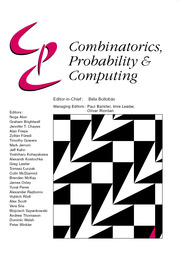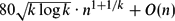Home
Hostname: page-component-8bbf57454-6xp8w Total loading time: 0.269 Render date: 2022-01-21T23:37:08.879Z Has data issue: true Feature Flags: { "shouldUseShareProductTool": true, "shouldUseHypothesis": true, "isUnsiloEnabled": true, "metricsAbstractViews": false, "figures": true, "newCiteModal": false, "newCitedByModal": true, "newEcommerce": true, "newUsageEvents": true }Combinatorics, Probability and Computing

A Bound on the Number of Edges in Graphs Without an Even Cycle

Published online by Cambridge University Press:  07 April 2016

Abstract

We show that, for each fixed k, an n-vertex graph not containing a cycle of length 2k has at most$80\sqrt{k\log k}\cdot n^{1+1/k}+O(n)$ edges.

MSC classification

Type
Paper
Information
Combinatorics, Probability and Computing , January 2017 , pp. 1 - 15

Access options

Get access to the full version of this content by using one of the access options below. (Log in options will check for institutional or personal access. Content may require purchase if you do not have access.)

References

 Benson, C. T. (1966) Minimal regular graphs of girths eight and twelve. Canad. J. Math. 18 10911094.CrossRefGoogle Scholar
 Blagojević, P. V. M., Bukh, B. and Karasev, R. (2013) Turán numbers for Ks,t -free graphs: Topological obstructions and algebraic constructions. Israel J. Math. 197 199214. arXiv:1108.5254.CrossRefGoogle Scholar
 Bondy, J. A. and Simonovits, M. (1974) Cycles of even length in graphs. J. Combin. Theory Ser. B 16 97105.CrossRefGoogle Scholar
 Brown, W. G. (1966) On graphs that do not contain a Thomsen graph. Canad. Math. Bull. 9 281285.CrossRefGoogle Scholar
 Erdős, P. (1938) On sequences of integers no one of which divides the product of two others and on some related problems. Inst. Math. Mech. Univ. Tomsk 2 7482.Google Scholar
 Erdős, P. (1964) Extremal problems in graph theory. In Theory of Graphs and its Applications (Proc. Sympos. Smolenice, 1963), Publ. House Czechoslovak Academy of Sciences, Prague, pp. 2936.Google Scholar
 Erdős, P. and Rényi, A. (1962) On a problem in the theory of graphs. Magyar Tud. Akad. Mat. Kutató Int. Közl. 7 623641.Google Scholar
 Füredi, Z. and Simonovits, M. (2013) The history of degenerate (bipartite) extremal graph problems. Bolyai Soc. Math. Stud. 25 169264. arXiv:1306.5167.CrossRefGoogle Scholar
 Füredi, Z., Naor, A. and Verstraëte, J. (2006) On the Turán number for the hexagon. Adv. Math. 203 476496.CrossRefGoogle Scholar
 Keevash, P. (2011) Hypergraph Turán problems. In Surveys in Combinatorics 2011, Vol. 392 of London Mathematical Society Lecture Note Series, Cambridge University Press, pp. 83139.CrossRefGoogle Scholar
 Kővari, T., Sós, V. T. and Turán, P. (1954) On a problem of K. Zarankiewicz. Colloquium Math. 3 5057.Google Scholar
 Lazebnik, F. and Ustimenko, V. A. (1995) Explicit construction of graphs with an arbitrary large girth and of large size. Discrete Appl. Math. 60 275284.CrossRefGoogle Scholar
 Mellinger, K. E. and Mubayi, D. (2005) Constructions of bipartite graphs from finite geometries. J. Graph Theory 49 110.CrossRefGoogle Scholar
 Pikhurko, O. (2012) A note on the Turán function of even cycles. Proc. Amer. Math. Soc. 140 36873692.CrossRefGoogle Scholar
 Sidorenko, A. (1995) What we know and what we do not know about Turán numbers. Graphs Combin. 11 179199.CrossRefGoogle Scholar
 Simonovits, M. (1968) A method for solving extremal problems in graph theory, stability problems. In Theory of Graphs: Proc. Colloq. Tihany 1966, Academic, pp. 279319.Google Scholar
 Turán, P. (1941) On an extremal problem in graph theory (in Hungarian). Mat. Fiz. Lapok 48 436452.Google Scholar
 Verstraëte, J. (2000) On arithmetic progressions of cycle lengths in graphs. Combin. Probab. Comput. 9 369373. arXiv:math/0204222.CrossRefGoogle Scholar
 Wenger, R. (1991) Extremal graphs with no C 4's, C 6's, or C 10's. J. Combin. Theory Ser. B 52 113116.CrossRefGoogle Scholar

15
Cited by

Send article to Kindle

Note you can select to send to either the @free.kindle.com or @kindle.com variations. ‘@free.kindle.com’ emails are free but can only be sent to your device when it is connected to wi-fi. ‘@kindle.com’ emails can be delivered even when you are not connected to wi-fi, but note that service fees apply.

Find out more about the Kindle Personal Document Service.

A Bound on the Number of Edges in Graphs Without an Even Cycle
Available formats
×

Send article to Dropbox

To send this article to your Dropbox account, please select one or more formats and confirm that you agree to abide by our usage policies. If this is the first time you use this feature, you will be asked to authorise Cambridge Core to connect with your <service> account. Find out more about sending content to Dropbox.

A Bound on the Number of Edges in Graphs Without an Even Cycle
Available formats
×

To send this article to your Google Drive account, please select one or more formats and confirm that you agree to abide by our usage policies. If this is the first time you use this feature, you will be asked to authorise Cambridge Core to connect with your <service> account. Find out more about sending content to Google Drive.

A Bound on the Number of Edges in Graphs Without an Even Cycle
Available formats
×
×